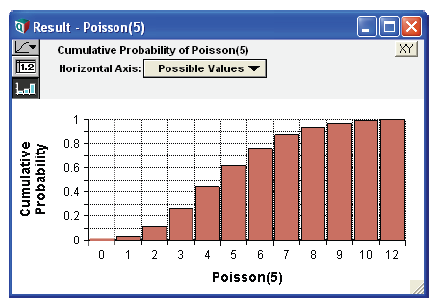# Probability density and mass graphs

When you select the Probability density as the uncertainty view for a continuous variable, it graphs the distribution as a Probability Density function. The height of the density shows the relative likelihood the variable has that value.Technically, the probability density of variable X, means the probability per unit increment of X. The units of probability density are the reciprocal of the units of X — if the units of X are dollars, the units of probability density are probability per dollar increment.

If you select Probability density as the uncertainty view for a discrete variable, it actually graphs the Probability Mass function — using a bar graph style to display the probability of each discrete value as the height of each bar.Similarly, if you choose the cumulative probability uncertainty view for a discrete variable, it actually displays the cumulative probability mass distribution as a bar graph. Each bar shows the cumulative probability that X has that value or any lower value.Is a distribution discrete or continuous? Almost always, Analytica can figure out whether a variable is discrete or continuous, and so choose the probability density or probability mass view as appropriate — so you don’t need to worry about it. If the values are text, it knows it must be discrete. If the numbers are integers, such as generated by Bernoulli, Poisson, binomial, and other discrete parametric distributions, it also assumes it is discrete.

Infrequently, a discrete distribution can contain numbers that are not integers, which it might not recognize as discrete, for example:

Chance Indiscrete := Poisson(4)*0.5

In this case, you can make sure it does what you want by specifying the domain attribute of the variable as discrete (or continuous). The next section on the domain attribute explains how.